Date: Mon, 30 Nov 1998 16:26:43 EST
To: QandQ@MathCentral.uregina.ca
Subject: area

How do you find the surface area of the 5 platonic solids, when they each have a volume of one cubic inch?

Thanks Rachel

Hi Rachel
In the Quandaries and Queries section of Math Central there is a note called What is the volume of a regular tetrahedron? that will give you enough information to answer this question for a tetrahedron.
In the book H.S.M. Coxeter, Regular Polytopes (second edition), The Macmillan Company, New York, 1948 there is a table that relates several properties of regular polytopes. Two of the columns in this table contain the information needed to solve your problem. Using Coxeter's notation that C is the volume, S is the surface area, 2L is the edge length and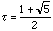, the appropriate information is contained in the table.

 Name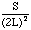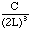Regular tetrahedron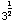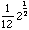Octahedron 2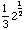Cube 6 1 Icosahedron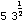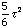Dodecahedron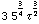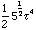Cheers,
Harley

Go to Math Central